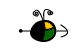Vapor Pressure Lowering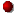Click here to review vapor pressure of pure liquids and solids.

The Macroscopic View

When a solute is added to a solvent, the vapor pressure of the solvent (above the resulting solution) is lower than the vapor pressure above the pure solvent.

The vapor pressure of the solvent above a solution changes as the concentration of the solute in the solution changes (but it does not depend on the identity of either the solvent or the solute(s) particles (kind, size or charge) in the solution).

Non-Volatile Solutes

Experimentally, we know that the vapor pressure of the solvent above a solution containing a non-volatile solute (i.e., a solute that does not have a vapor pressure of its own) is directly proportional to the mole fraction of solvent in the solution. This behavior is summed up in Raoult's Law:

Psolvent = XsolventPosolvent

where:

Psolvent is the vapor pressure of the solvent above the solution,
Xsolvent is the mole fraction of the solvent in the solution, and
Posolvent is the vapor pressure of the pure solvent.

Note that

• when Xsolvent = 1 (pure solvent), Psolvent = Posolvent, and
• when Xsolvent < 1 (solute(s) present), Psolvent < Posolvent (i.e., the vapor pressure of the solvent above the solution is lower than the vapor pressure above the pure solvent).

The following graph shows the vapor pressure for water (solvent) at 90oC as a function of mole fraction of water in several solutions containing sucrose (a non-volatile solute). Note that the vapor pressure of water decreases as the concentration of sucrose increases.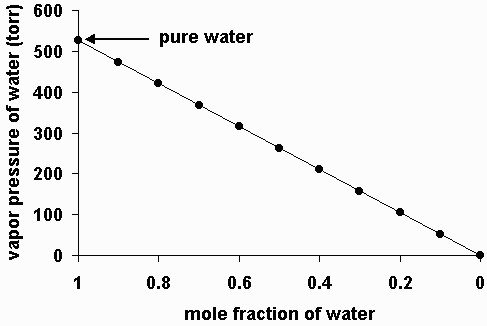Volatile Solutes

A volatile solute (i.e., a solute that has a vapor pressure of its own) will contribute to the vapor pressure above a solution in which it is dissolved. The vapor pressure above a solution containing a volatile solute (or solutes) is equal to the sum of the vapor pressures of the solvent and each of the volatile solutes.

Psolvent = XsolventPosolvent

Psolute = XsolutePosolute

Psolution = Psolvent + Psolute + ... = XsolventPosolvent + XsolutePosolute + ...

The Microscopic View

The figure below shows a microscopic view of the surface of pure water. Note the interface between liquid water (below) and water vapor (above).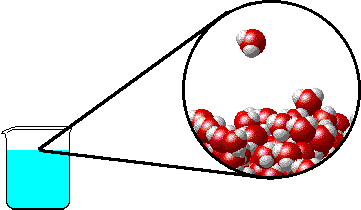Non-Volatile Solutes

The figures below illustrate how the vapor pressure of water is affected by the addition of the non-volatile solute, NaCl.

Note that:

• there are fewer water molecules in the vapor (i.e., lower vapor pressure) above the NaCl solution than in the vapor above pure water, and
• there are no sodium ions or chloride ions in the vapor above the NaCl solution.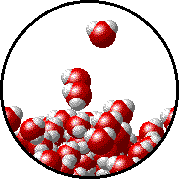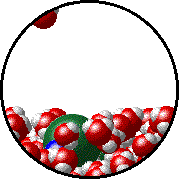Pure water - microscopic view. 1.0 M NaCl solution - microscopic view.Note that the ionic solid, NaCl, produces Na+ ions (blue) and Cl- ions (green) when dissolved in water.

Volatile Solutes

The figures below illustrate how the vapor pressure of liquid xenon is affected by the addition of the volatile solute, liquid krypton.

Note that:

• there are fewer xenon atoms in the vapor (i.e., lower vapor pressure) above the solution than in the vapor above pure liquid xenon, and
• there are krypton atoms in the vapor above the solution (i.e., both krypton and xenon contribute to the vapor pressure above the solution).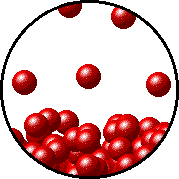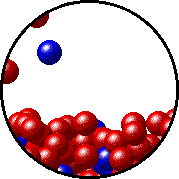Pure liquid xenon - microscopic view. Krypton-xenon solution - microscopic view (krypton atoms are shown in blue).